# Numerical simulation of fish meat freezing with considering temperature-dependent thermal properties

Numerical simulation of fish meat freezing with considering temperature-dependent thermal properties

Farzad SepahvandiHamid M. Heravi Seyed R. Saleh

Corresponding Author Email:
Page:
75-81
|
DOI:
https://doi.org/10.18280/ijht.350110
|
Accepted:
|
Published:
31 March 2017
| Citation

OPEN ACCESS

Abstract:

Applying the computational fluid dynamic model (CFD), which can simulate the effect of freezing on meat properties, will help to save in time and cost. Especially when predicting the time for freezing the product with certain thermal properties. In this paper, CFD models created in two-dimensional in order to simulate the cooling process in terms freezing tunnel of refrigerator. Obtained Numerical results were compared with experimental data. The geometric of fish meat has been modeled and required time for freezing each of them has been obtained and compared with together.  The storage temperature of each of meat was found from tables and handbooks and applied in simulation. The level of freezing in different times has been presented in temperature and freezing mass fraction counters. This condition validates by comparing the experimental and obtained data for fish meat. In addition, it is noticeable that freezing temperature for various meats is different from each other. In addition, the geometric of beef has been modeled and required time for freezing each of them has been obtained and compared with together.  The level of freezing in different times has been presented in temperature counters.

Keywords:

Numerical Simulation, Fish meat, Freezing, Heat Transfer

1. Introduction

The quality of meat and food value is affected by five factors: transferring, cooling, processing, packing and storing. The way of storing of meat products is very crucial factor which must be attended during transporting. In order to maintain freshness during transport, controlling temperature of refrigerator is important factor. The logical cooling causes long refrigerator life of meat (Lauzon et al. ). Among the two phase phenomenon, researchers concentrate and study on freezing process due to contribute in wide spread of research area as: metal processing, food cooling, Casting process, envirmental engineering, ice accumulation in airplane wings, melting of snow and Ice land, cooling of land, increasing the volume of soil due to Glacial, freezing of marine and aerial structures, transmission line of fluids and energy storing system.  The freezing process was named as motion boundary problems which also introduce as Stephan problems (issue). This process happens with heat conduction along with phase changing and the main characteristic of freezing is changing of phase concurrent with releasing the latent heat due to changing the phase. Heat transferring with phase change is non-linear problems .the most paper which published in the mass and heat transfer area is related to cooling of products and very few of these models have been studied for food production [3-5]. Aldousari  investigated the progress of pressure in limited pipe, which contains water while ice is growing and presented some ways for holding the pressure constant for avoiding the pipes bursting during maintenance. Two-dimensional heat transfer and laminar flow freezing inside the pipe with Small diameter was analyzed numerically with fluent software by Conde et al.  which shows good agreement with experimental data. Seong and Sik  works on the Theory and conducted experimental research on the effect of pipes diameter and cooling length to the forming of ice ball in freezing process with liquid Nitrogen during maintenance, in this regard, they found out the importance of these two factors on the forming ice. Richardson et al.  called the freezing phenomenon as a valuable process because of Local freezing pipes, which is noticeable in industry for solving repair and maintenance of pipelines.

Lomauro et al.  reported statistics due to humidity distribution constant of cow’s meat. Motarjemi  also represented humidity distribution constant of mince of cow’s meat at various temperature and humidity. Merts et al.  introduced and discover novel approach for evaluating the drying curve of one sample of meat and found some technical points. Radford et al.  used drying technic for determination humidity distribution constant in their survey.

In the recent years, Lattice-Boltzmann method has been used to simulate phase change problems [14-15].

In the present study, the effect of freezing CSIRO approach on fish will be analyzed numerically by considering time. For increasing the accuracy of research, thermal properties of meat have been considered as a function of temperature.

2. Numerical Simulation of Fish Meat Freezing

For predicting the required time to freeze the foods, information for their thermal properties is essential. In this part, white fish freezing has been studied. Freezing temperature according to Margeirsson et al. [16-18] reports is -0.92 oC and we used this value in this work. When the meat of fish cooled near to freezing temperature, phase changing in fish muscle occurs with forming ice crystals. According to Fikkin, each fish meat contains 80.3% of water. Table 1 shows the dependence of heat conduction coefficient (k) and specific heat capacity (Cp) at constant pressure to temperature.

Table 1. Heat conduction coefficient (k) and specific heat capacity (Cp) in different temperatures for fish meat (Zueco et al.  and Johnston et al. )

 T (oC) -4 -0.92 0 5 10 k (W/(mK)) 1.361 1.302 0.43 0.43 0.43 Cp (kJ/(kgK)) 15.11 223 4.144 3.641 3.683

The value of density (ρ) has been considered 1054 kg/m3 according to . The results in table 1 show the dependence of physical properties of fish to temperature especially at below -0.92 oC. Figure 1 shows the considered fish which will be simulated and studied in Fluent and will be compared with previous works. One two-dimensional model has been simulated with finite volume and the obtained results are compared with experimental results at various times.

## 1.png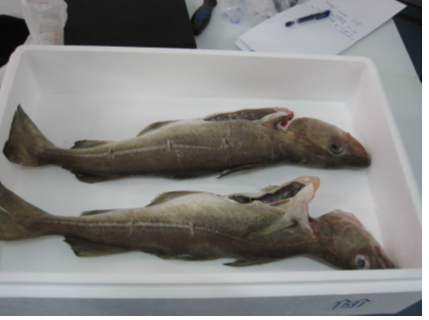Figure 1. The simulated fish at present paper

3. Geometry

The schematic of mentioned fish has shown in figure 2. The dimension is in Mm. The Gambit software has been used for creating geometry and meshing. In model related to fish, triangular two-dimensional cells have been utilized which is represented in figure 3. Due to freezing dependence to temperature, the problem study in unsteady condition. Therefore the time step (Δt) is considered 0.1 second and used pressure based for solving the analysis. For solving the governor equations, SIMPLE algorithm has been proposed.  For the surface of fish, flow motion assumed. For discretizing the equation Second Order Upwind has been used and considers the residual equal to 10-6. For introducing the meat properties as specific heat capacity and thermal conduction coefficient which is function of temperature, one UDF written and added to software.

## 2.png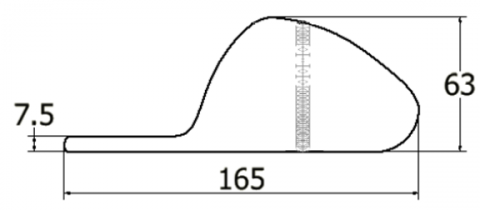Figure 2. Two-Dimensional model of simulated fish

## 111111111.pngFigure 3. Model of two-dimensional fish

4. Energy Equation

Generally, the problems which explained the Transient phenomenon, can convert to partial difference equations which satisfy the boundary and initial condition. According to energy conservation equation and cooling low of Fourier for one differential control volume and presume that fish is cylinder, with this assumption the energy equation must be solved as following as:

$u\frac{\partial T}{\partial x}+v\frac{\partial T}{\partial y}=\alpha \frac{{{\partial }^{2}}T}{\partial {{y}^{2}}}$          (1)

which is the thermal diffusion coefficient. As k and Cp are function of temperature but the value of ρ is constant.

5. Calculation of Thermal Properties

In this paper, freezing process of software models solved with different assumption and then numerical data compared with experimental data. The fallowing assumption, which have been applied:

1. Software model with assuming constant value for food thermal properties. For example these properties could be obtained from averaging the thermal properties at two stages; fish not frozen and completely frozen .

2. Software model which thermal properties are function of temperature, used from experimental data. By accessing to these data or with creating linkage between thermal properties and temperature, can be used from this approach and algorithm for all foods. For instance: for calculation thermal conduction coefficient (k), and specific heat (Cp) of fish can be referred to Zhao et al.  equation.

${{C}_{p}}={{C}_{pf}}+({{n}_{wo}}-{{M}_{b}}{{n}_{s}})({{T}_{w,f}}-{{T}_{i,f}}){{H}_{o}}/({{T}_{w,f}}-T)\,\,,\,\,If\text{ }T<{{T}_{i,f}}$         (2)

${{C}_{p}}={{C}_{po}}\,\,,\,\,If\text{ }T>{{T}_{i,f}}$         (3)

$k={{k}_{f}}+({{k}_{0}}-{{k}_{f}})({{T}_{w,f}}-{{T}_{i,f}})/({{T}_{w,f}}-T)\,\,,\,\,If\text{ }T<{{T}_{i,f}}$            (4)

$k={{k}_{o}}\,\,,\,\,~If\text{ }T>{{T}_{i,f}}$           (5)

6. Results

For the case which initial temperature of fish is 5 oC and the freezer temperature is -35C the obtained results presented in table 2. By referring to this table, it can be observed motion boundary condition has more accurate result than constant temperature boundary condition.

Table 2. The comparison between present numerical and experimental results

 Model Boundary condition Δt (s) Present numerical freezing time (hour) Experimental freezing time (hour)  Numerical Model with constant thermal properties (case 1) Constant temperature 180 0.3 5 300 0.3 5 Motion boundary 180 0.55 5 300 0.58 5 Numerical Model with variable thermal properties (case 2) Constant temperature 180 2.3 5 300 1.92 5 Motion boundary 180 4.9 5 300 5.08 5

Table 2 shows that there is noticeable difference in the result by considering various boundary conditions and this effect shows thermal properties with low accuracy in obtaining times of freezing. In the freezing of porous food as meat, water evaporates from surface of meat and during freezing water pass from inner layer. During this phenomenon, water sublimate at surface of meat, which is not noticeable and compare with heat transfer phenomenon, mass transfer happens at outer region of meat and is negligible. Furthermore, in dense foods, because of low rate of humidity diffusion, the portion of penetration in heat transfer is negligible . In this research, the chosen tissue is fish and non-porous.

The figures 4 and 5 show the counters of temperature and freezing fraction at various times (second) for variable properties. As clear in the figures, the center of foods in the stage of freezing, spend   more time in critical region which has constant value of temperature. In the beginning of freezing, temperature increasing is tangible due to release of the latent heat of crystallization and crystal formation. Also temperature gradient and freezing fraction due to air motion is equal from outer layer to center. It can be concluded, the tail of fish due to low thickness freezes suddenly.

## 4.pngFigure 4. Temperature counters at: (a) t = 0.5 hr, (b) t = 2 hr, (c) t = 3.5 hr, (d) t = 5 hr

## 5.pngFigure 5. Freezing fraction counters at: (a) t = 0.5 hr, (b) t = 2 hr, (c) t = 3.5 hr, (d) t = 5 hr

Also the effect of initial temperature on freezing time has been investigated and observed there is no significant difference in initial temperature with air flow motion in freezing of fish. This issue has been shown in figure 6. Our analysis shows that time freezing difference for freezer with -35 oC with the -25 oC is almost one hour.

## 6.jpgFigure 6. Effect of initial fish temperature on freezing time at T0=5, 20, 40oC

With regards to figure 6, in initial times, the center of material is not affected with outside temperature variation.  By more temperature decreasing of surface and subsequently temperature exchanging with inside the fish, the temperature of center will be decreased quickly up to initial freezing of fish. Up to this point, heat transfer to center of fish is due to heat penetration. After this phenomenon, because of phase changing of inside water, temperature is constant for a period of time. The process of phase changing which accompany with heat releasing is time-consuming action. However, by going across the fish due to fast heat transfer, the range of phase changing will be decreased. According to our results in this analysis, insoluble materials in humidity of fish cause to decrease the freezing point to less than water freezing point. In initial freezing, some amount of water freeze and the concentration of remaining solution will increase. With regard to this matter, the freezing point of unfrozen part of fish decreases and this phenomenon will dominate the freezing phenomenon. After passing required time and complete freezing of center of fish as figure 6 shows, temperature decreasing starts again until the temperature equilibrium with surrounding fluid and center of fish. The main mechanism for heat transfer in this period is heat penetration. By going the end of this process, fish and surrounding fluid will isotherm and by decreasing the gradient of temperature, the rate of heat transfer in this range will decrease. Figure 7 presents the amount of released heat of fish for the first 360 sec. Although this value is negative but the absolute value has been considered.

## 7.jpgFigure 7. Fish released heat at first 350 seconds

In order to see the effect of the fish thickness on the freezing speed, three thickness in the z axis direction for fish are investigated including 10, 15 and 20 cm. In figures 8-10 results from this study is shown. As it is apparent, increase in thickness increases the time required for freezing the fish meat and it is clear by comparison of figures.

## 8.png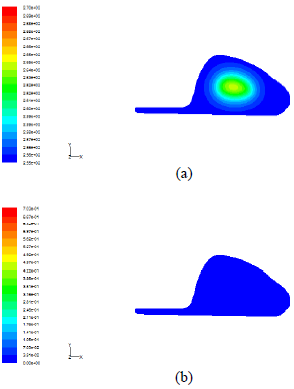Figure 8. Freezing process of fish for the thickness of 10 cm in the z axis direction after 1100 s. (a) Static Temperature Contours, (b) Freezing fraction counters

## 9.pngFigure 9. Freezing process of fish for the thickness of 15 cm in the z axis direction after 1100 s. (a) Static temperature contours, (b) Freezing fraction counters

## 10.png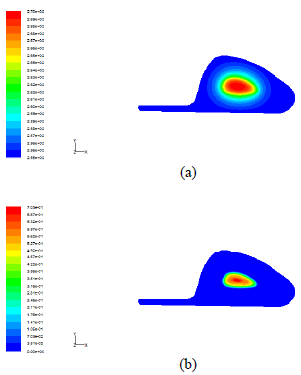Figure 10. Freezing process of fish for the thickness of 20 cm in the z axis direction after 1100 s. (a) Static temperature contours, (b) Freezing fraction counters

A two-dimensional material that is cooled by free convection stream is shown in figure 11. Type of material is beef and its dimensions are considered 10×10cm. Solution area including the food material is discretized by a non-uniform network. According to Figure 8, grids become fine in the wall direction. Thermo-physical properties of material depend on temperature except than fluid density that following Boussinesq approximations. For simplicity, the non-dimension time is used for this case as following as:

$\tau =\frac{t\alpha }{{{L}^{2}}}$           (6)

α is thermal diffusion coefficient and L is the length of material i.e. 10 cm.

## 11.jpgFigure 11.  Two-dimensional CFD model of beef created by software

Figures 12-14 display Temperature and freezing fraction counters at different times for the two-dimensional model. During the time of freezing, cold layers penetration into the food material can be seen. According to these figure 9 in the first moments of freezing, the core of material still has not been affected by the outside temperature and therefore its temperature does not change. With the passage of time and the heat exchange with the temperature, core temperature began to decrease. This heat transfer into the food is the heat diffusion to the food surface.

## 12.png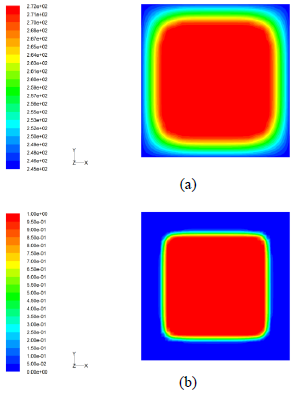Figure 12. Beef Freezing Process after Dimensionless Time of τ = 5. a) Static Temperature Contour, (b) Freezing fraction counters

## 13.pngFigure 13. Beef Freezing Process after Dimensionless Time of τ = 25. a) Static Temperature Contour, (b) Freezing fraction countere

## 14.png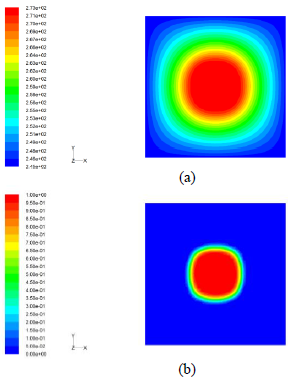Figure 14. Beef Freezing Process after Dimensionless Time of τ = 40. a) Static Temperature Contour, (b) Freezing fraction counters

7. Conclusion

In this article, heat transfer modeling of food product during freezing due to fluid motion on the surface of fish has been studied. Two types of meat (fish and beef) with different geometries were simulated and the time required to freeze any of them were obtained and compared. Storage temperature of each of meat was extracted from tables and handbooks and applied in the simulations. The freezing level has been shown in different mass fraction and temperature counters during different times. This condition has been validated by comparison between experimental and obtained data for fish and also software settings in this way were used for beef meat. It should be noted that freezing temperatures are different for different meats. Two-dimensional model was simulated for beef. Three-dimensional model was used to determine effect of thickness on temperature distribution for fish meat. One of the novelties of this research, applying two numerical approaches with constant thermal properties (Case 1) and variable thermal properties to temperature (case 2), the boundary conditions considered for two cases are constant temperature and convection heat transfer. The obtained result show that there is noticeable difference between experimental and numerical data by applying constant thermal properties and constant temperature boundary condition and this efficacy indicates the inaccuracy of obtained freezing time by considering of thermal properties with low accuracy. In addition, temperature increasing observed again in initial freezing due to releasing of latent heat of crystallization and formation of ice crystals. Furthermore, temperature gradient and freezing fraction from surface to center because of air motion is the same. Also the effect of initial temperature of fish on the time of freezing was studied and obtained data shows the freezing point of fish due to being insoluble materials in humidity of fish is less than water. In initial freezing, some amount of water freeze and the concentration of remaining solution will increase. With repetition this matter, the freezing point of unfrozen part of fish decreases and this phenomenon will dominate the freezing phenomenon. In addition, for the beef freezing it was seen that in the first moments of freezing, the core of material still has not been affected by the outside temperature and therefore its temperature does not change. With the passage of time and the heat exchange with the temperature, core temperature began to decrease. This heat transfer into the food is the heat diffusion to the food surface.

References

 Lauzon H.L., Margeirsson B., Sveinsdóttir K., Guðjónsdóttir M., Karlsdóttir M.G., Martinsdóttir E., (2010). Overview on fish quality research. Impact of fish handling, processing, storage and logistics on fish quality deterioration. Matís report.

 Puri, V.M., Anantheswaran R.C. (1993). The finite-element method in food processing: a review, Journal of Food Engineering, Vol. 19, pp. 247-274.

 Salvadori V.O., Mascheroni R.H., De Michelis A. (1996). Freezing of strawberry pulp in large containers: experimental determination and prediction of freezing times, International Journal of Refrigeration, Vol. 19, pp. 87-94.

 Tocci A.M., Mascheroni R.H. (1995). Numerical models for simulation of the simultaneous heat and mass transfer during freezing and storage,  International Communications in Heat Mass Transfer, Vol. 22, pp. 251-260.

 Tocci A.M., Mascheroni R.H. (1995). Heat and mass transfer coefficients during the refrigeration, freezing and storage of meats, meat products and analogues,  Journal of Food Engineering, Vol. 13, pp. 147-160.

 Aldousari S. (2007). Utilization of phase change for sealing and pressurization of tubular elements, J KAAU: Engineering Sciences, Vol. 15, No. 2, pp. 89-107.

 Conde R., Parra M.T., Castro F.J., Villafruela M., Rodríguez M.A., Méndez C. (2004). Numerical model for two-phase solidification problem in a pipe,  Applied Thermal Engineering, Vol. 24, No. 17-18, December, pp. 2501-2509.

 Seong J., Sik Y. (2002). Numerical analysis of phase change and natural convection phenomena during pipe freezing process, ASME, Vol. 448, No. 2, pp. 145-149.

 Richardson R.N., Bowen R.J.,  Sharman A. (2003). Accelerated pipe freezing in non-circular sections,   Chemical Engineering Research & Design: Transactions of the Institution of Chemical Engineers Part A, Vol. 81, No. 4, pp. 46-77.

 Lomauro C.J., Bakshi A.S., Labuza T. P. (1990). Moisture transfer properties of dry and semimoist foods,  Journal of Food Science, Vol. 55, pp. 218-223.

 Motarjemi Y. (1998). A Study of some Physical Properties of Water in Foodstuffs, PhD Thesis, Department of Food Engineering, Lund University, Lund, Sweden.

 Merts I., Lovatt S.J., Lawson C.R. (1998). Diffusivity of moisture in whole muscle meat measured by a drying curve method, IIR Proceedings Series, Refrigeration Science and Technology, Sofia, Bulgaria.

 Radford R.D., Herbert C., Lovett D.A. (1976). Chilling of meat- A mathematical model for heat and mass transfer, Proc. Comm. C2 Meeting, IIR Bull. Annex 1, pp. 323-330.

 Huang X.P., Chen Z.Q., Shi J. (2016). Simulation of solid-liquid phase transition process in aluminum foams using the Lattice Boltzmann method, International Journal of Heat and Technology, Vol. 34, No. 4, pp. 694-700. DOI: 10.18280/ijht.340420

 Mahmoudi A., Mejri I. (2015). Analysis of conduction-radiation heat transfer with variable thermal conductivity and variable refractive index: application of the Lattice Boltzmann method, International Journal of Heat and Technology, Vol. 33, No. 1, pp. 1-8. DOI: 10.18280/ijht.330101

 Margeirsson B., Lauzon H.L., Þorvaldsson L., Árnason S.V., Arason S., Valtýsdóttir K.L., Martinsdóttir, E. (2010). Optimized chilling protocols for fresh fish, Matís report, Vol. 54, pp.10-18.

 Margeirsson B., Pálsson H., Popov V., Gospavic R., Arason S., Sveinsdóttir K., Þór Jónsson M. (2012). Numerical modelling of temperature fluctuations in superchilled fish loins packaged in expanded polystyrene and stored at dynamic temperature conditions, International Journal of Refrigeration, Vol. 35, No. 5, pp. 1073-1080.

 Margeirsson B., Gospavic R., Pálsson H., Arason S., Popov V. (2011). Experimental and numerical modeling comparison of thermal performance of expanded polystyrene and corrugated plastic packaging for fresh fish, International Journal of Refrigeration, Vol. 34, No. 2, pp. 573–585,.

 Zueco J., Alhama F., Fernandez C.G. (2004). Inverse determination of the specific heat of foods, Journal of Food Engineering, Vol. 64, No. 3, pp. 78-91.

 Johnston W.A., Nicholson A.R., Stroud G.D. (1994). Freezing and refrigerated storage in fisheries, Journal of Food Engineering, Vol. 9, pp. 87-94.

 Jahnston W.A., Nicholson F.J., Roger A., Stroud G.D. (1944). Freezing and refrigerated storage in fisheries, FAO, Vol. 2, No.1, 103-113.

 Zhao Y., Edward K., Cormae C. (1998). Computer simulation on onboard chilling and freezing of Albacore Tuna, Journal of Food Science, Vol. 63, No. 5, pp.751-755.

 Vassilis G. (1992). Transport Phenomena of Foods and Biological Materials, CRC Press.

 Tuan Pham Q. (2006). Modeling heat and mass transfer in frozen foods: a review, International Journal of Refrigeration, Vol. 28, pp. 1-13.